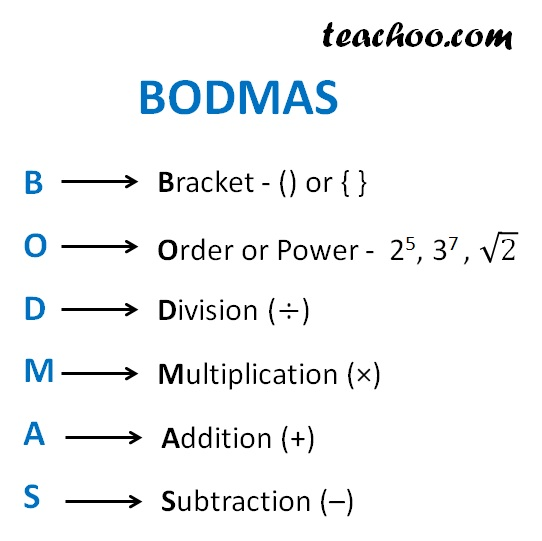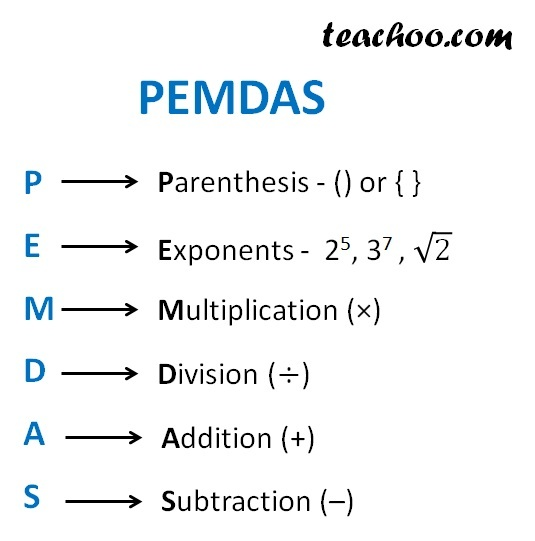Definitions

Chapter 1 Class 6 Knowing our Numbers
Concept wise

Suppose we want to calculate

2 × 4 + 3 – (8 – 1)

How do we do it?

Do we multiply first?

Or Subtract?

We use BODMAS ruleBODMAS stands for B racket, O rder, D ivision, M ultiplication, A ddition and S ubtraction

Let’s get back to our question

2 × 4 + 3 – (8 – 1)

In BODMAS

• We first solve B racket
• Then O rder
• Then D ivision & M ultiplication are at the same level, so we go from left to right and solve them.
• A ddition and S ubtraction are at the same level, so we go from left to right and solve them

### 2 × 4 + 3 – (8 – 1)

2 × 4 + 3 – (8 – 1)

= 2 × 4 + 3 – 7

= 8 + 3 – 7

= 11 – 7

= 4

Note :

Here we first solve B racket – (8 – 1)

Then there is no O rder,

So, we solve D ivision & M ultiplication left to right – 2 × 4

And then, we solve A ddition and S ubtraction left to right – 8 + 3 and 11 – 7

Let’s take some more examples

### 15 ÷ 3 × 1 ÷ 5

15 ÷ 3 × 1 ÷ 5

= 15 ÷ 3 × 1 ÷ 5

= 15/3 × 1 ÷ 5

= 5 × 1  ÷ 5

= 5 ÷ 5

= 5/5

= 1

Note :

Here we have no B racket, or O rder

So, we solve D ivision & M ultiplication left to right – first 15 ÷ 3 , then 5 × 1 and then 5 ÷ 5

And then, A ddition and S ubtraction are not there

### 48 ÷ 2 (9 + 3)

48 ÷ 2 (9 + 3)

= 48 ÷ 2 × (9 + 3)

= 48 ÷ 2 × 12

= 48/2 × 12

= 24 × 12

= 288

Note :

Here we first solve B racket – (9 + 3)

Then there is no O rder,

So, we solve D ivision & M ultiplication left to right – First 48 ÷ 2, then 24 ×  12

And then, A ddition and S ubtraction are not there

### 10² – 4 ÷ 2

10² – 4 ÷ 2

= 10²  – 4 ÷ 2

= 100 –  4 ÷  2

= 100 – 4/2

= 100 – 2

= 98

Note :

Here, there is no B racket

Then, we solve O rder – 10 2 ,

Then, we solve D ivision & M ultiplication left to right – 4 ÷ 2

And then, A ddition and S ubtraction left to right – 100 – 2

### 14 ÷ 7  + 4 × 8 – 2 3

14 ÷ 7  + 4 × 8 – 2 3

= 14 ÷ 7  + 4 × 8 – 8

= 2 + 4 × 8 – 8

= 2 + 32 – 8

= 34 – 8

= 26

Note :

Here, there is no B racket

Then, we solve O rder – 2 3 ,

Then, we solve D ivision & M ultiplication left to right – first 14 ÷ 7, then 4 × 8

And then, A ddition and S ubtraction left to right – first 7 + 32, then 39 – 8

### (4 × 3 2 – 2 3 ÷ 4) + 10 – 5

(4 × 3 2 2 3 ÷ 4) + 10 – 5

= (4 × 9 – 8 ÷ 4) + 10 – 5

= (36 – 8 ÷ 4 ) + 10 – 5

= (36 – 8/4) + 10 – 5

= (36 – 2) + 10 – 5

= (34) + 10 – 5

= 34 + 10 – 5

= 44 – 5

= 39

Note :

Here, there is a B racket, so we solve it first

In bracket,

We first solve O rder – 3 2 , 2 3 ,

Then, we solve D ivision & M ultiplication left to right – first 4 × 9, then 8 ÷ 4

And then, A ddition and S ubtraction left to right – first 36 – 4 in bracket, then 32 + 10, 42 – 5

## PEMDAS

BODMAS in some Regions is called PEMDAS

PEMDAS stands for P arenthesis, E xponents, M ultiplication, D ivision, A ddition, S ubtractionIn PEMDAS

• We first solve P arenthesis
• Then E xponents
• Then M ultiplication & D ivision are at the same level, so we go from left to right and solve them
• A ddition and S ubtraction are at the same level, so we go from left to right and solve them

You can remember it by saying

P lease  E xcuse  M D ear  A unt  S ally

Learn in your speed, with individual attention - Teachoo Maths 1-on-1 Class

### Transcript

Bracket - () or { } Order or Power - 25, 37 , √2 Division (÷) Multiplication (×) Addition (+) Subtraction (–) Parenthesis - () or { } Exponents - 25, 37 , √2 Multiplication (×) Division (÷) Addition (+) Subtraction (–)